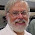Tuesday, November 22, 2011

Deconstructing vertex corrections

Ultimately much of quantum many-body theory concerns calculating correlation functions which are measurable. For example, the conductivity can written as a current-current correlation function [Kubo formula]. The simplest approximation neglects vertex corrections and just calculates the "bubble" diagram consisting of the product of Green's functions.

What are vertex corrections? When do they matter? What sort of robust or general results are available about them?

Many people, including myself, often just ignore them. I fear this is partly motivated by difficulty rather good scientific criteria.

Below are a few things I am slowly learning, re-learning, and digesting.

Migdal showed that for the electron-phonon interaction the vertex corrections are small due to the smallness of the ratio of the electronic mass to the nuclear mass [alternatively the ratio of the speed of sound to the Fermi velocity].
But, Migdal's argument breaks down for an electron-magnon interaction.

Neglecting vertex corrections is equivalent to making the relaxation time approximation (RTA) when solving the Boltzmann equation. Then the quasi-particle lifetime equals the transport lifetime because one ignores dependence of the scattering rate on momentum transfer. Below is some helpful text from a review by Kontani:

....we have to take the Current Vertex Correction [CVC] into account correctly, which is totally dropped in the Relaxation Time Approximation [RTA]. In interacting electron systems, an excited electron induces other particle– hole excitations by collisions. The CVC represents the induced current due to these particle–hole excitations. The CVC is closely related to the momentum conservation law, which is mathematically described using the Ward identity [28–31]. In fact, Landau proved the existence of the CVC, which is called backflow in the phenomenological Fermi liquid theory, as a natural consequence of the conservation law . The CVC can be significant in strongly correlated Fermi liquids owing to strong electron–electron scattering.

For specific types of interactions Ward identities allow one to relate the vertex function to derivatives of the self energy. Mahan's book (Section 8.1.3) discusses this in detail.

In the limit of infinite dimensions [in which Dynamical Mean-Field Theory (DMFT)] becomes exact, vertex corrections can be neglected.

In a recent PRB, Bergeron, Hankevych, Kyung, and Tremblay calculated the optical conductivity for the Hubbard model at the level of a two-particle self-consistent approach, including the constraint of the f-sum rule. They found that at "high" temperatures (T > 0.2t) vertex corrections did not matter much, but were significant at lower temperatures near a quantum critical point.

1 comment:

1.Concerning vertex corrections for fermions in the presence of d=2 antiferromagnetic fluctuations, we find that it is in the pseudogap regime that the qualitative changes brougth about by vertex corrections are most important. In the pseudogap regime, the antiferromagnetic correlation length is larger than the thermal de Broglie wavelength. Then the effective interaction between dressed quasiparticles mediated by antiferromagnetic fluctuations has strong momentum dependence. This leads to important vertex corrections that can modify the temperature dependence of the resistivity. Vertex corrections do have an influence also at the quantum critical point.